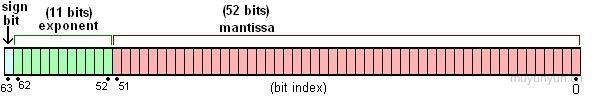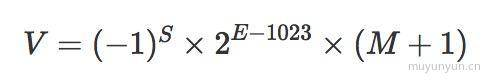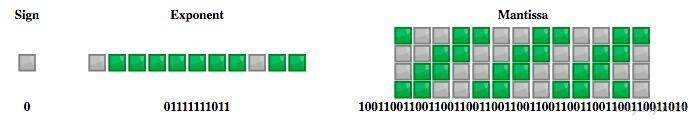Time Flying# 探寻 JavaScript 精度问题

### 十进制小数转为二进制小数方法

①. 针对整数部分 173, 采取除 2 取余, 逆序排列;

173 / 2 = 86 ... 186 / 2 = 43 ... 043 / 2 = 21 ... 1   ↑21 / 2 = 10 ... 1   | 逆序排列10 / 2 = 5 ... 0    |5 / 2 = 2 ... 1     |2 / 2 = 1 ... 01 / 2 = 0 ... 1

②. 针对小数部分 0.8125, 采用乘 2 取整, 顺序排列;

0.8125 * 2 = 1.625  |0.625 * 2 = 1.25    | 顺序排列0.25 * 2 = 0.5      |0.5 * 2 = 1         ↓

③. 将前面两部的结果相加, 结果为 10101101.1101;

### 小心, 二进制小数丢失了精度！

0.1 * 2 = 0.20.2 * 2 = 0.4 // 注意这里0.4 * 2 = 0.80.8 * 2 = 1.60.6 * 2 = 1.20.2 * 2 = 0.4 // 注意这里, 循环开始0.4 * 2 = 0.80.8 * 2 = 1.60.6 * 2 = 1.2...

### 推导 0.1 + 0.2 为何等于 0.30000000000000004• sign(符号): 占 1 bit, 0 表示正, 1 表示负;
• exponent(指数): 占 11 bit, 表示范围;
• mantissa(尾数): 占 52 bit, 表示精度, 多出的末尾如果是 1 需要进位;0.1 转化为二进制为 0.0001100110011..., 用科学计数法表示为 1.100110011... x 2^(-4), 根据上述公式, S0(1 bit), E-4 + 1023, 对应的二进制为 01111111011(11 bit), M1001100110011001100110011001100110011001100110011010(52 bit, 另外注意末尾的进位), 0.1 的存储示意图如下:0.1 + 0.2 即 2^(-4) x 1.1001100110011001100110011001100110011001100110011010 与 2^(-3) x 1.1001100110011001100110011001100110011001100110011010 之和

// 计算过程0.000110011001100110011001100110011001100110011001100110100.0011001100110011001100110011001100110011001100110011010
// 相加得0.01001100110011001100110011001100110011001100110011001110

0.01001100110011001100110011001100110011001100110011001110 转化为十进制就是 0.30000000000000004。验证完成!

### JavaScript 的最大安全数是如何来的

Math.pow(2, 53) === Math.pow(2, 53) + 1 // true

Math.pow(2, 53) 竟然与 Math.pow(2, 53) + 1 相等！这是因为 Math.pow(2, 53) + 1 已经超过了尾数的精度限制(53 bit), 在这个例子中 Math.pow(2, 53)Math.pow(2, 53) + 1 对应了同一个双精度浮点数。所以 Math.pow(2, 53) 就不是安全数了。

### 业务中碰到的精度问题以及解决方案

1.针对大数的整数可以考虑使用 BigInt 类型(目前在 stage 3 stage4 阶段)；

2.使用 bigNumber, 它的思想是转化成 string 进行处理, 这种方式对性能有一定影响;

3.可以考虑使用 long.js, 它的思想是将长度为 64 位的 long 类型值转化成两个精度为 32 位的双精度类型的值。

4.针对小数的话可以使用 number-precision, 该库将小数转为整数后再作处理;